Richard Melson

July 2006

Rankine & Entropy

Rankine

### 1820 - 1872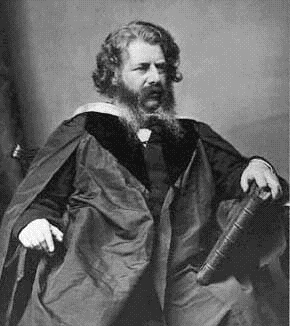Rankine cycle

The Rankine cycle is a thermodynamic cycle. Like other thermodynamic cycles, the maximum efficiency of the Rankine cycle is given by calculating the maximum efficiency of the Carnot cycle. It is named after William John Macquorn Rankine, a Scottish polymath.

This article will deal with the Rankine cycle from an engineering point of view.

Processes of the Rankine cycle

There are four processes in the Rankine cycle, each changing the state of the working fluid. These states are identified by number in the diagram above.

• Process 4-1: First, the working fluid is pumped (ideally isentropically) from low to high pressure by a pump. Pumping requires a power input (for example mechanical or electrical).

• Process 1-2: The high pressure liquid enters a boiler where it is heated at constant pressure by an external heat source to become a superheated vapor. Common heat sources for power plant systems are coal, natural gas, or nuclear power.

• Process 2-3: The superheated vapor expands through a turbine to generate power output. Ideally, this expansion is isentropic. This decreases the temperature and pressure of the vapor.

• Process 3-4: The vapor then enters a condenser where it is cooled to become a saturated liquid. This liquid then re-enters the pump and the cycle repeats.

## Description

Rankine cycles describe the operation of steam heat engines commonly found in power generation plants. In such vapour power plants, power is generated by alternately vaporizing and condensing a working fluid (in many cases water, although refrigerants such as ammonia may also be used).

The working fluid in a Rankine cycle follows a closed loop and is re-used constantly. Water vapour seen billowing from power plants is evaporating cooling water, not working fluid. (NB: steam is invisible).

## Equations

Each of the first four equations are easily derived from the energy and mass balance for a control volume. The fifth equation defines the thermodynamic efficiency of the cycle as the ratio of net power output to heat input.

## Real Rankine cycle (non-ideal)

In a real Rankine cycle, the compression by the pump and the expansion in the turbine are not isentropic. In other words, these processes are non-reversible and entropy is increased during the two processes (indicated in the figure as ?S). This somewhat increases the power required by the pump and decreases the power generated by the turbine. It also makes calculations more involved and difficult.

## Variations of the basic Rankine cycle

Two main variations of the basic Rankine cycle are used in modern practice.

### Rankine cycle with reheat

In this variation, two turbines work in series. The first accepts vapor from the boiler at high pressure. After the vapor has passed through the first turbine, it re-enters the boiler and is reheated before passing through a second, lower pressure turbine. Among other advantages, this prevents the vapor from condensing during its expansion which can seriously damage the turbine blades.

### Regenerative Rankine cycle

The regenerative Rankine cycle is so named because after emerging from the condenser (possibly as a subcooled liquid) the working fluid is heated by steam tapped from the hot portion of the cycle. This increases the [[]] of heat addition which in turn increases the thermodynamic efficiency of the cycle.

## References

There are four processes in the Rankine cycle, each changing the state of the working fluid. These states are identified by number in the diagram above.

• Process 4-1: First, the working fluid is pumped (ideally isentropically) from low to high pressure by a pump. Pumping requires a power input (for example mechanical or electrical).

• Process 1-2: The high pressure liquid enters a boiler where it is heated at constant pressure by an external heat source to become a superheated vapor. Common heat sources for power plant systems are coal, natural gas, or nuclear power.

• Process 2-3: The superheated vapor expands through a turbine to generate power output. Ideally, this expansion is isentropic. This decreases the temperature and pressure of the vapor.

• Process 3-4: The vapor then enters a condenser where it is cooled to become a saturated liquid. This liquid then re-enters the pump and the cycle repeats.

## Description

Rankine cycles describe the operation of steam heat engines commonly found in power generation plants. In such vapour power plants, power is generated by alternately vaporizing and condensing a working fluid (in many cases water, although refrigerants such as ammonia may also be used).

The working fluid in a Rankine cycle follows a closed loop and is re-used constantly. Water vapour seen billowing from power plants is evaporating cooling water, not working fluid. (NB: steam is invisible until it comes in contact with cool, saturated air, at which point it condenses and forms the white billowy clouds seen leaving cooling towers).

## Real Rankine cycle (non-ideal)

In a real Rankine cycle, the compression by the pump and the expansion in the turbine are not isentropic. In other words, these processes are non-reversible and entropy is increased during the two processes (indicated in the figure as ?S). This somewhat increases the power required by the pump and decreases the power generated by the turbine. It also makes calculations more involved and difficult.

## Variations of the basic Rankine cycle

Two main variations of the basic Rankine cycle are used in modern practice.

### Rankine cycle with reheat

In this variation, two turbines work in series. The first accepts vapor from the boiler at high pressure. After the vapor has passed through the first turbine, it re-enters the boiler and is reheated before passing through a second, lower pressure turbine. Among other advantages, this prevents the vapor from condensing during its expansion which can seriously damage the turbine blades.

### Regenerative Rankine cycle

The regenerative Rankine cycle is so named because after emerging from the condenser (possibly as a subcooled liquid) the working fluid is heated by steam tapped from the hot portion of the cycle. This increases the average temperature of heat addition which in turn increases the thermodynamic efficiency of the cycle.

## References

• Moran & Shapiro 'Fundamentals of Engineering Thermodynamics'

(ISBN 0471274712)

Variables

###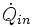heat input rate (energy per unit time)mass flow rate (mass per unit time)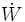mechanical power used by or provided to the system (energy per unit time)

eta (Greek scientific symbol)

thermodynamic efficiency of the process (power used for turbine per heat input, unitless)

h1,h2,h3,h4

these are the "specific enthalpies" at indicated points on the T-S diagram

## Equations

Each of the first four equations are easily derived from the energy and mass balance for a control volume. The fifth equation defines the thermodynamic efficiency of the cycle as the ratio of net power output to heat input.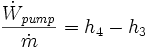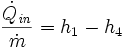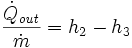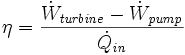Rankine & Energy

July 27, 2006